# Is equal

Is equal following terms?Result

#### Solution:Leave us a comment of example and its solution (i.e. if it is still somewhat unclear...):Be the first to comment!## Next similar examples:

1. Unknown number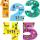Determine the unknown number, which double of its fourth square is equal the fifth its square.
2. The percentages in practiceIf every tenth apple on the tree is rotten it can be expressed by percentages: 10% of the apples on the tree is rotten. Tell percent using the following information: a. in June rained 6 days b, increase worker pay 500 euros to 50 euros c, grabbed 21 fro
3. Powers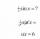Is true for any number a,b,c equality:? ?
4. Unknown number x5% from an unknown number equals the number 26. What is the unknown number?
5. Binomials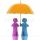To binomial ? add a number to the resulting trinomial be square of binomial.
6. Unknown number 17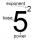Milada said, I am thinking of a number such that I evaluate expression x1/3, the value of the expression would be 5. Which number Milada thinking?
7. Proof I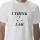When added to the product of two consecutive integers larger one, we get square larger one. Is this true or not?
8. PowersExpress the expression ? as the n-th power of the base 10.
9. One halfOne half of ? is: ?
10. Expressions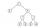Find out value of expressions if a = -1, b =2: x=b - 2a - ab y=a3 - b2 - 2ab z=a2 b3 - a3 b2 w=a + b + a3 - b2
11. PercentsHow many percents is 900 greater than the number 750?
12. Linear systemSolve this linear system (two linear equations with two unknowns): x+y =36 19x+22y=720
13. Center traverseIt is true that the middle traverse bisects the triangle?
14. Highway repairThe highway repair was planned for 15 days. However, it was reduced by 30%. How many days did the repair of the highway last?
15. Hotel roomsIn the 45 rooms, there were 169 guests, some rooms were three-bedrooms and some five-bedrooms. How many rooms were?
16. PersonsPersons surveyed:100 with result: Volleyball=15% Baseball=9% Sepak Takraw=8% Pingpong=8% Basketball=60% Find the average how many like Basketball and Volleyball. Please show your solution.
17. TheatroTheatrical performance was attended by 480 spectators. Women were in the audience 40 more than men and children 60 less than half of adult spectators. How many men, women and children attended a theater performance?